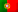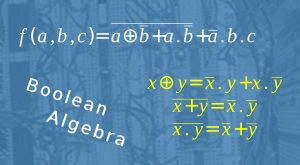Home > Technology > Computer Science > Computer Architecture > Boolean Algebra and Logical Functions

# Boolean Algebra and Logical Functions

Chapter II – Logical SystemsBoolean Algebra and Logical Functions

Chapter I – Numeral Systems

1. Logical or boolean operations
2. Boolean Algebra and Logical Functions
3. Logic Gates

More articles under development…

## Introduction

In the previous article, we studied the truth tables and the common operations we can perform. Now, let’s take a look at Boolean Algebra and logical functions. In fact, in the real world, what we have in the world of digital circuits are circuits with some inputs and one or more outputs, whose values will depend on the input values.

Let’s say we have a controller for the motor of a lift and we want to activate an output (set to 1) to start the motor to make the lift to go up, whenever the following conditions, or states, are met:

• One button is pressed for an upper floor
• The lift is stopped
• The lift’s door is closed
• There are others… But we are just giving a very simple example.

Keeping this simple, we can think of a function “F” for our output and the inputs A, B and C, where:

• “A” tells if a valid button was pressed;
• “B” indicates if the lift is currently moving;
• “C” if the door is open.

According to this information, we can now write our function as:

f(a,b,c) = a  . b . c

And this is a logical function we can read as “F is equal to A AND (NOT B) AND (NOT C). As we only have AND operators, we can easily conclude that F will only be true (F = 1) when:

• A = 1 (a valid button is being pressed)
• B = 0 (The lift is not moving)
• C = 0 (The lift’s door is not open)

The following table shows us the common operators we find in these functions and their respective logical function.

 A . B , A x B, AB A AND B A + B A OR B A ⊕ B A XOR B A NOT A

Let’s take another example:

f(a,b,c) = a + (b ⊕ c)

Please note that we have one operation between brackets, and here we have to respect that as well. The following table will help us to determine all possible values for F for all the inputs.

ABCCB ⊕ CF = A + (B ⊕ C)
000111
001000
010100
011011
100111
101001
110101
111011

As you can see, we used all the possible combinations for A, B and C, and we also determined “NOT C”. Then we determined the results for BC ( B XOR (NOT C)) and finally we determined the final result with the OR between the input A and the last XOR results. With this, we’ve just found all possible values for out function F.

Another important note! We are working in the digital world, with binary numbers, so the domain on any boolean function is always the set {0.1} and the co-domain will be contained in this set as well.

## Boolean Algebra Laws

Let’s now take a look at some laws we find in Boolean Algebra. We will find some similar laws with our common algebra but remember we are in the binary world. Also, like multiplication and division, the logical operator AND has priority against the operator OR, but remember that the symbols used here, like “+”, “x” or “.”,  to not correspond to addition and multiplication but to the logical operators OR and AND.

Laws in Boolean Algebra
Associativity of ANDA . (B . C) = (A . B) . C
Associativity of ORA + (B + C) = (A + B) + C
Commutativity of ANDA . B = B . A
Commutativity of ORA + B = B + A
Distributivity of AND over ORA . (B + C) = (A . B) + (A . C)
Distributivity of OR over ANDA + (B . C) = (A + B) . (A + C)
Absorption in ANDA . (A + B) = A
Absorption in ORA + (A . B) = A
Identity for ANDA . 1 = A
Identity for ORA + 0 = A
Annihilator for ANDA . 0 = 0
Annihilator for ORA . 1 = 1
Complementation in ANDA . A = 0
Complementation in ORA + A = 1
Idempotence of ANDA x A = A
Idempotence of ORA + A = A
Double negationA = A

We may find some awkward laws like the distributivity of OR over AND, which does not exist in common algebra if we think about distributivity of addition over multiplication. But again, we need to remember that we are not in common algebra and this kind of laws are true in boolean algebra and all these laws can be proven.

Now, regarding XOR, we can also apply some of the previous laws, specially the commutativity and the associativity. Let’s see a couple of specific cases and some interesting transformations:

• A ⊕ A = 0 ; A ⊕ 0 = A
• A ⊕ A = 1 ; A ⊕ 1 = A (remember a XOR example from the previous article)
• A ⊕ B = (A . B) + (A . B)
• A ⊕ B = (A . B) . (A + B)

Let’s demonstrate the penultimate transformation using the truth tables:

ABA ⊕ BBA . BAA . BA . B + A . B
00010100
01100111
10111001
11000000

Try to demonstrate the last transformation!

## De Morgan’s Laws

Very well known in the world of digital circuits, De Morgan’s Laws are very useful to find equivalent circuits and / or to help to simplify logical functions.

As leis de De Morgan (ou apenas leis de Morgan) são muito conhecidas no mundo dos circuitos digitais. Com elas, facilmente podemos encontrar circuitos equivalentes e/ou simplificar funções lógicas.

According to De Morgan’s Laws, we have these two very important relations:

• A . B = A + B
• A + B = A . B

We are going to demonstrate the first law using truth tables:

ABA . BA . BABA + B
0001111
0101101
1001011
1110000

Try to demonstrate the second law!

References: